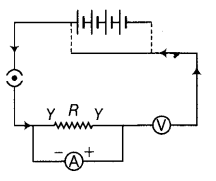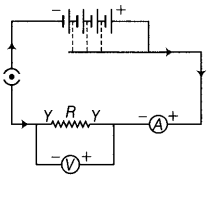# A child has drawn the electric circuit to study Ohm's law as shown in figure

A child has drawn the electric circuit to study Ohm’s law as shown in figure. His teacher told that the circuit diagram needs correction. Study the circuit diagram and redraw it after making all corrections.The circuit diagram is not correct because of the following reasons:
(i) Ammeter is connected in parallel with R and voltmeter connected in series with it in contrary of the fact that ammeter should be connected in series and voltmeter in parallel. This is so because same current flows in series and same voltage exist in parallel combination.
(ii) The current is drawn from negative terminal and enter into the battery at possible terminal which is not possible in one battery circuit.
(iii) Cells are not connected in series combination in the battery of the circuit.
The correct diagram is given as follow: